# Agricultural Engineering- AG 2012 GATE Paper (Practice Test)

## 65 Questions MCQ Test GATE Past Year Papers for Practice (All Branches) | Agricultural Engineering- AG 2012 GATE Paper (Practice Test)

Description
Attempt Agricultural Engineering- AG 2012 GATE Paper (Practice Test) | 65 questions in 180 minutes | Mock test for GATE preparation | Free important questions MCQ to study GATE Past Year Papers for Practice (All Branches) for GATE Exam | Download free PDF with solutions
QUESTION: 1

Solution:
QUESTION: 2

Solution:
QUESTION: 3

### Choose the most appropriate alternative from the options given below to complete the following sentence: The administrators went on to implement yet another unreasonable measure, arguing that the measures were already ___ and one more would hardly make a difference.

Solution:
QUESTION: 4

Choose the most appropriate alternative from the options given below to complete the following sentence:

To those of us who had always thought him timid, his ___ came as a surprise.

Solution:
QUESTION: 5

The arithmetic mean of five different natural numbers is 12. The largest possible value among the numbers is

Solution:
QUESTION: 6

Q. 6 - Q. 10 carry two marks each.

Two policemen, A and B, fire once each at the same time at an escaping convict. The probability that A hits the convict is three times the probability that B hits the convict. If the probability of the convict not getting injured is 0.5, the probability that B hits the convict is

Solution:
QUESTION: 7

The total runs scored by four cricketers P, Q, R, and S in years 2009 and 2010 are given in the following table: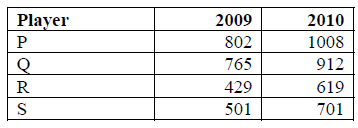The player with the lowest percentage increase in total runs is

Solution:
QUESTION: 8

If a prime number on division by 4 gives a remainder of 1, then that number can be expressed as

Solution:
QUESTION: 9

Two points (4, p) and (0, q) lie on a straight line having a slope of 3/4. The value of (p – q) is

Solution:
QUESTION: 10

In the early nineteenth century, theories of social evolution were inspired less by Biology than by the conviction of social scientists that there was a growing improvement in social institutions. Progress was taken for granted and social scientists attempted to discover its laws and phases.

Which one of the following inferences may be drawn with the greatest accuracy from the above passage?

Social scientists

Solution:
QUESTION: 11

Q. 11 – Q. 35 carry one mark each.

The matrix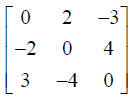is

Solution:
QUESTION: 12

The line y = x −1 can be expressed in polar coordinates (r,θ ) as

Solution:
QUESTION: 13

The type of pump used in forced water cooling system of a tractor engine is

Solution:
QUESTION: 14

Which one of the following statements is NOT appropriate regarding cone index

Solution:
QUESTION: 15

The draft and total power requirement of a rotary cultivator operating in concurrent mode as compared to a spring tyne cultivator of equal cutting width under the same operating conditions, respectively are

Solution:
QUESTION: 16

The soil erodibility factor needs to be determined for use in the universal soil loss equation. The length, in m and slope, in % of the experimental plot to be used for this purpose, respectively are

Solution:
QUESTION: 17

The difference between Fore Bearing and Back Bearing of a traverse line is

Solution:
QUESTION: 18

A pumping device that combines the advantages of both centrifugal and reciprocating pumps is known as

Solution:
QUESTION: 19

If ν is the kinematic viscosity of air – water vapour mixture and DAB is the mass diffusivity of water vapour in air then the ratio ν/DAB is known as

Solution:
QUESTION: 20

Work index in size reduction can be obtained by multiplying Bond’s energy constant with

Solution:
QUESTION: 21

The tangent line to y = f (x) at the point (xo , yo ) , assuming f ′(x) ≠ 0, intersects the x axis at

Solution:
QUESTION: 22

Approximate percentage of scores that fall within ±σ (standard deviation) of the mean in a normal distribution is

Solution:
QUESTION: 23

The integrating factor of the differential equation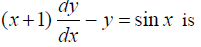Solution:
QUESTION: 24

The constituent of producer gas which occupies the highest percentage by volume and helps in increasing its overall calorific value is

Solution:
QUESTION: 25

During field operation, the shank of a tractor drawn rigid tyne sweep type cultivator is mainly subjected to

Solution:
QUESTION: 26

A slider is moving on a straight link at a sliding velocity of 0.5 m s−1. The straight link is pivoted at one end and makes angular movement at a rate of 1.0 rad s−1. Coriolis acceleration of the slider in m s−2 is

Solution:
QUESTION: 27

The power developed and the exhaust gas temperature of a diesel engine compared to a spark ignition engine of the same size and running at the same speed respectively, are

Solution:
QUESTION: 28

In a semi-modular outlet, the discharge

Solution:
QUESTION: 29

The relationship between outflow Q in m3 s−1 and storage S in m3 for an emergency spillway in a reservoir is Q = S/4000. Inflow, outflow and storage are assumed to be zero at time t = 0. If the inflow rate is 300 m3 s−1 at the end of t = 3 hours, the outflow rate in m3 s−1 is

Solution:
QUESTION: 30

A trapezoidal grassed waterway is constructed along a longitudinal gradient of 4%. If the crosssectional area of flow is 1.52 m2, wetted perimeter is 12.5 m and Manning’s n for the waterway is 0.04 m−1/3 s, the flow through the waterway in m3 s−1 is

Solution:
QUESTION: 31

A single acting reciprocating pump discharges 3.5 litres of water per second at 40 rpm. The pump has a piston diameter of 150 mm and a stroke of 300 mm. The percentage slip is

Solution:
QUESTION: 32

A pair of parallel glass panes, each of 3 mm thickness traps 2 mm layer of stagnant air. Thermal conductivities of glass and air are 0.5 and 0.02 W m−1 K −1, respectively. If the film heat transfer coefficient of air is 10 W m−2 K−1, then Biot Number is

Solution:
QUESTION: 33

Two small parallel plane square surfaces, each measuring 4 mm × 4 mm are placed 0.5 m apart (centre to centre) with 30° angle between the radial distance and both the surface normals. The view factor between the two surfaces is

Solution:
QUESTION: 34

Tomato catsup with 10 Pa sconsistency coefficient and 0.8 flow behaviour index is flowing in a pipe. Generalized coefficient of viscosity of catsup, in Pa sn is

Solution:
QUESTION: 35

A packed bed of 480 kg solid particles having particle size of 0.15 mm and density of 800 kg m−3 is fluidized using air at 25 °C and 1 atmospheric pressure. If the cross section of the empty bed is 0.45 m2 and voidage at minimum fluidizing condition is 0.5, then the minimum height of the fluidized bed, in m is

Solution:
QUESTION: 36

Q. 36 to Q. 65 carry two marks each

The value of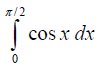using trapezoidal rule with two equal intervals is

Solution:
QUESTION: 37

A tractor power take-off (PTO) driven stationary peg tooth type wheat thresher operating at a cylinder speed of 540 rpm requires a torque of 250 Nm at PTO. The minimum net engine power required, in kW is

Solution:
QUESTION: 38

A border strip of 8 × 250 m is being irrigated by a border stream of 50 lps. The infiltration capacity of the soil is 25 mm h−1 (assumed to be constant throughout the period of irrigation). The average depth of the advancing sheet of water over the land is 70 mm. The time required to irrigate the border strip, in minutes, will be

Solution:
QUESTION: 39

Decimal reduction times for Bacillus subtilis are 37 s and 12 s at temperatures of 120 °C and 125 °C, respectively. The temperature rise, in °C, necessary to reduce the first value of decimal reduction time at 120 °C by a factor of 10 is

Solution:
QUESTION: 40

A tall silo having height to diameter ratio of 2 is holding 480 tons wheat of bulk density 960 kg m−3. The angle of internal friction for wheat is 25° and for wheat and wall surface is 24°. Applying Airy formula, the maximum lateral pressure in kPa at the bottom of the bin section is

Solution:
QUESTION: 41

The eigenvalues of the matrix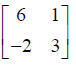are

Solution:
QUESTION: 42

If f '(x) = ex and f (0) = 5 , then from Mean Value Theorem, the value of f (1) lies between

Solution:
QUESTION: 43

The inverse Laplace Transform of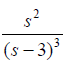can be written as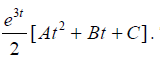The values of A,
B and C, respectively are

Solution:
QUESTION: 44

A two wheel drive tractor, weighing 15.84 kN with a wheel base of 2160 mm, has the static weight divided between the front and rear axles in the ratio of 30 : 70 on a horizontal level surface. The hitch point is at a height of 700 mm from the ground and at a horizontal distance of 120 mm to the rear side from the center of the rear axle. Pull acts at an angle of 12° downwards from the horizontal. The maximum pull in kN, when the front wheels would just start rising from the ground is

Solution:
QUESTION: 45

A horizontal axis drag type wind mill with square blades and a horizontal axis lift type wind mill with airfoil section blades having same rotor size are installed at a height of 10 m above the ground. The average wind speed is 25 km h−1. The maximum power coefficient for drag type and lift type wind mills is 0.148 and 0.593, respectively. If the maximum power extracted by drag type wind mill is 5 kW, the corresponding power extracted by lift type wind mill, in kW is

Solution:
QUESTION: 46

The thresher of a wheat combine harvester has an optimal throughput capacity of 2400 kg (crop) per hour. The harvester has a forward velocity of 4.5 km h−1. Sample tests have revealed that the yield of crop in the field is 3000 kg (grain) per ha. Grain to straw ratio is 60 : 40. If the above throughput is to be maintained, the width of cut of the harvester in m, neglecting turning losses, is

Solution:
QUESTION: 47

A regime channel carrying a discharge of 25 m3 s−1 is designed using Lacey’s regime theory. The side slope of the channel is ½ H : 1 V, and Lacey’s silt factor is unity. The bottom width and depth of flow in the channel, in m, respectively are

Solution:
QUESTION: 48

Flow is taking place through a layered soil system, having two homogeneous soils M and N, as shown in the figure. The head lost in soil N is 20 times the head lost in soil M.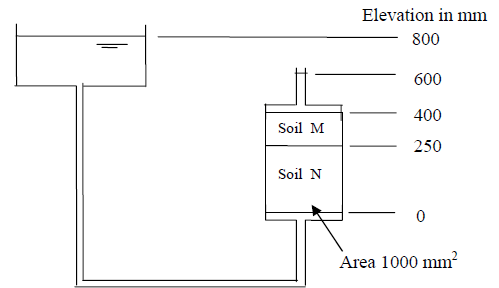If the permeability of soil M is 3 × 10 −4 mm s−1, the permeability of soil N, in mm s−1, will be

Solution:
QUESTION: 49

A trapezoidal canal, having a bottom width of 5.0 m and a side slope of 1 H : 1 V, is carrying a discharge of 20 m3 s−1. The critical depth, in m, is

Solution:
QUESTION: 50

A 200 mm well fully penetrates a confined aquifer. After a long period of pumping at a rate of 1400 litres per minute, the drawdowns in the observation wells located at 25 m and 40 m from the pumping well are found to be 2.6 m and 1.9 m, respectively. The transmissivity of the aquifer in m2 day−1 is

Solution:
QUESTION: 51

Tile drains have to be installed in an agricultural land having soil permeability of 2.3 × 10−3 mm s−1. An impermeable stratum exists at 3.2 m below the land surface, and it is desired to keep the water level at least 1.0 m below the land surface. The average discharge of the drainage system is 2.0 mm day−1. If the tile drains are planned to be placed at 1.5 m below the land surface, the drain spacing in m, assuming the equivalent depth to be the same as the tile depth, is

corrct answer will be updated soon. Temperary mark A

Solution:
QUESTION: 52

It is proposed to construct bench terraces on a 10% hill slope. If the batter slope is ½ H : 1 V, the percentage area that will be lost for cultivation due to bench terracing is

Solution:
QUESTION: 53

Air at 70 °C and 0.015 humidity ratio is cooled adiabatically by spraying water. The final temperature of the air is 55 °C. Specific heat capacities of dry air and water vapour are 1.005 and 1.88 kJ kg−1 K−1, respectively and latent heat of vapourization of water at 0 °C is 2501.7 kJ kg−1. The absolute humidity of the outlet air, in kg water vapour per kg dry air is

Solution:
QUESTION: 54

Final mass flow rate of osmotically dehydrated cherries after finish drying from 18% dry basis moisture content to 11.5% wet basis moisture content is 5000 kg per hour. The dryer efficiency is 70%, latent heat of vapourization is 2345 kJ kg–1, specific heat of air is 1.005 kJ kg−1 K–1, drying temperature is 50 °C and the specific volume of ambient air at 25 °C is 0.866 m3 kg−1. The necessary air flow requirement for the drying system in m3 min−1 is

Solution:
QUESTION: 55

A single effect vacuum evaporator has 100 tubes of 25 mm diameter. One thousand kg feed of milk per hour with 15% TS is concentrated to 20% TS in the evaporator. Film heat transfer coefficients on either sides of the tube are 5000 and 800 W m−2 K−1. Thermal conductivity of 1.5 mm thick SS tubes is 15 W m−1 K−1. Latent heat of vapourization under vacuum is 2309 kJ kg −1. For 10 °C temperature difference across the tube wall, the height of each tube, in m is

Solution:
QUESTION: 56

One thousand units of mixed fruit bar, each weighing 100 g with a surface area of 0.01 m2, are frozen from 70 °C molten mass condition to −20 °C frozen storage condition within 3 hours. The specific heat capacity values of the bar are 3.6 kJ kg−1 K −1 and 1.97 kJ kg −1 K −1 before and after freezing point (0 °C) respectively. If the latent heat of crystallization is 250 kJ kg −1, the cooling capacity of the refrigeration unit required in tons of refrigeration is

Solution:
QUESTION: 57

In a disc clutch, the inside and outside radii of the clutch plate are 50 and 100 mm, respectively. If the axial force exerted on the disc is 4 kN, the maximum pressure experienced by the clutch plate in N mm-2 under uniform wear conditions is

Solution:
QUESTION: 58

Common Data Questions

Common Data for Questions 58 and 59:

A diesel engine running in dual fuel mode with diesel as pilot fuel and producer gas as primary fuel produces 3.5 kW at rated engine speed and is coupled directly to a generator for producing electricity. The amount of diesel and producer gas consumed per hour is 460 ml and 12.5 m3, respectively.

Assuming calorific value of diesel and producer gas as 35280 and 3.97 MJ m−3, respectively, the brake thermal efficiency of the engine in percentage is

Solution:
QUESTION: 59

A diesel engine running in dual fuel mode with diesel as pilot fuel and producer gas as primary fuel produces 3.5 kW at rated engine speed and is coupled directly to a generator for producing electricity. The amount of diesel and producer gas consumed per hour is 460 ml and 12.5 m3, respectively.

If generator efficiency is 90%, the maximum electricity produced, in kW is

Solution:
QUESTION: 60

Common Data for Questions 60 and 61:

The hourly discharge observations at the mouth of a watershed due to 2 cm excess rainfall during 0 to 1 h and 3 cm excess rainfall during 1 to 2 h are given in the table below. Assume a constant base flow of 1 m3 s−1.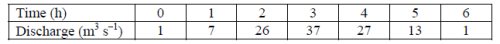The area of the watershed, in km2 is

Solution:
QUESTION: 61

The hourly discharge observations at the mouth of a watershed due to 2 cm excess rainfall during 0 to 1 h and 3 cm excess rainfall during 1 to 2 h are given in the table below. Assume a constant base flow of 1 m3 s−1.The peak of 1 h unit hydrograph in m3s−1 for the watershed and its time of occurrence in h, respectively are

Solution:
QUESTION: 62

Soybean is to be planted with a precision planter that meters 54 seeds per revolution of the metering disc powered from a ground wheel of diameter 490 mm. The desired plant population is 44800 per ha with a row to row spacing of 0.75 m. The germination percentage is 84. The planter is to be operated at 2.5 km h−1 with a 10% skid of ground wheel.

The angular speed of ground wheel in rpm is

Solution:
QUESTION: 63

Soybean is to be planted with a precision planter that meters 54 seeds per revolution of the metering disc powered from a ground wheel of diameter 490 mm. The desired plant population is 44800 per ha with a row to row spacing of 0.75 m. The germination percentage is 84. The planter is to be operated at 2.5 km h−1 with a 10% skid of ground wheel.

The angular speed ratio of metering disc to ground wheel for obtaining the desired plant population is

Solution:
QUESTION: 64

A 1 hp motor is used for running a dual cylinder reciprocating compressor of a refrigeration system based on R-134a refrigerant having 185 kJ kg−1 cooling capacity. COP of the system is 4.2 and overall efficiency of the compressor is 80%. Specific volume of the refrigerant vapour at suction temperature is 0.15 m3 kg−1. The compressor with bore diameters of 40 mm each runs at 1440 rpm.

The mass flow rate of the refrigerant in kg min−1 is

Solution:
QUESTION: 65

A 1 hp motor is used for running a dual cylinder reciprocating compressor of a refrigeration system based on R-134a refrigerant having 185 kJ kg−1 cooling capacity. COP of the system is 4.2 and overall efficiency of the compressor is 80%. Specific volume of the refrigerant vapour at suction temperature is 0.15 m3 kg−1. The compressor with bore diameters of 40 mm each runs at 1440 rpm.

The compressor stroke length in mm is

Solution:Use Code STAYHOME200 and get INR 200 additional OFF Use Coupon Code